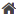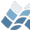NV5 GEOSPATIAL SOFTWARE>  Docs Center  >  Libraries  >  JBIU  >  BIHARMONIC_INTERP### BIHARMONIC_INTERP

BIHARMONIC_INTERP

## Name

BIHARMONIC_INTERP

## Purpose

Performs biharmonic interpolation for a function defined on a 2D grid.

Math

## Calling Sequence

Result = BIHARMONIC_INTERP(Z, X, Y, Xout, Yout)

## Inputs

Z: 2D array containing the values of the function defined at each
X,Y grid point. The first dimension of Z must have the same length
as X and the second dimension must have the same length as Y.
X: Vector containing the x-locations of the grid points at which
the function is defined.
Y: Vector containing the y-locations of the grid points at which
the function is defined.
Xout: A vector of x-locations at which the function is to be interpolated.
Yout: A vector of y-locations at which the function is to be interpolated.

## Outputs

A vector containing the value of the function biharmonically
interpreted at each Xout,Yout pair. The output will have the
same number of elements as Xout and Yout.

## Example

IDL> xgrid = [1.,2.]
IDL> ygrid = [2.,5.]
IDL> zvals = [ [10.,2.], [25.,15.] ]
IDL> PRINT, BIHARMONIC_INTERP(zvals, xgrid, ygrid, [1.3], [3.])
13.2745

## Procedure

Biharmonic interplation of Z(X,Y) is equivalent to bilinear
interpolation on the grid Z( log(X), log(Y) ), which is in fact
how it is calculated.

## Modification History

Written by: Jeremy Bailin
11 June 2008 Public release in JBIU

© 2023 NV5 Geospatial |  Legal